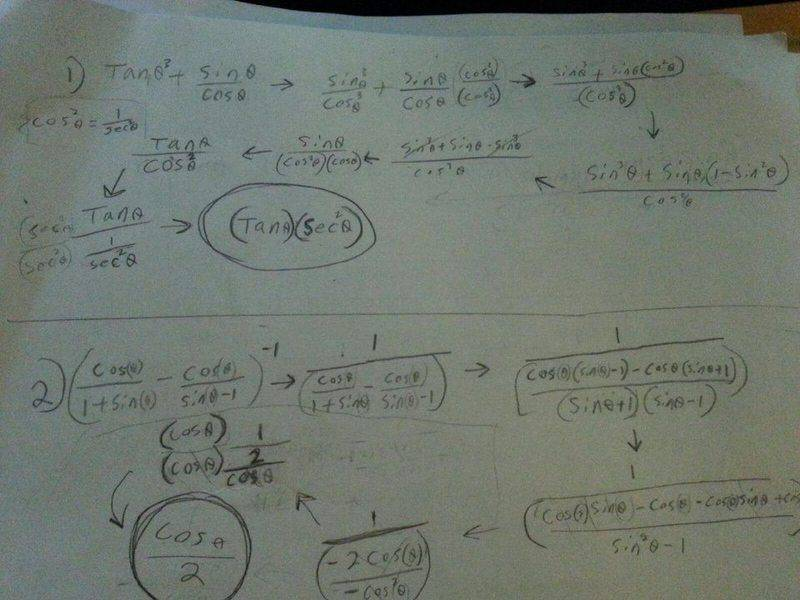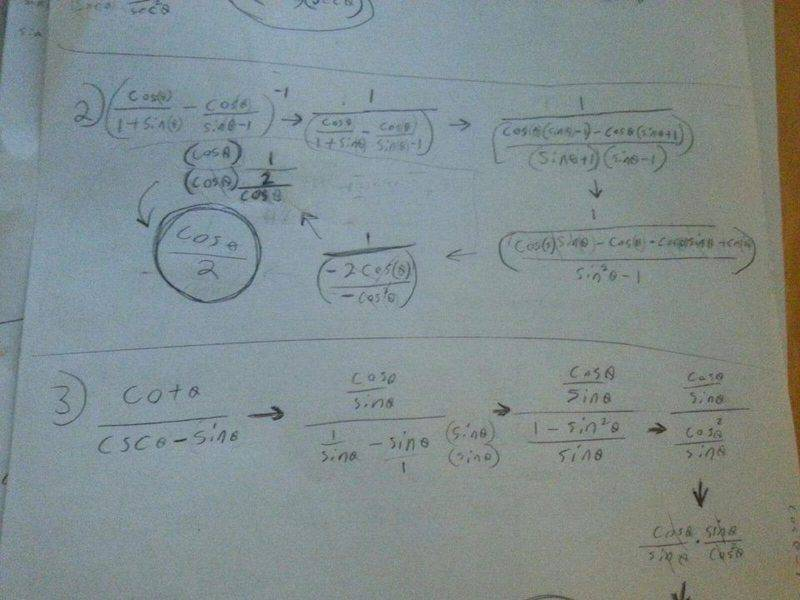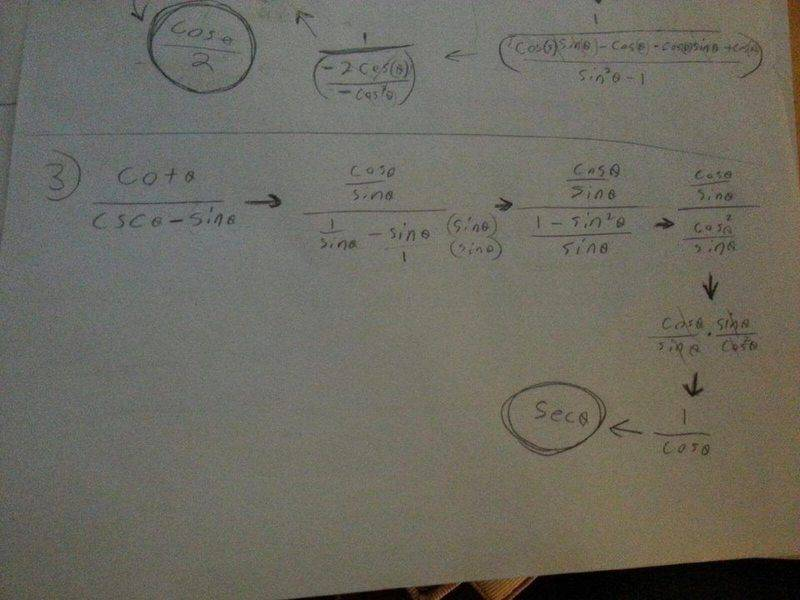# Trig Identities

Cosmic-Kat
I have re-post this forum as I should have paid closer attention to rules. I apologized for that.

## Homework Statement

1) The expression tan^3 θ + sinθ/cosθ is equal to:

(a) cot θ (b) tan θ sec^2 θ (c) tan θ (d) sin θ tan θ (e) tan θ csc^2 θ

2) Simplify (cos θ/1+ sin θ - cosθ/sinθ-1)^-1

(a) cos θ/2 (b) 2sec θ (c) 2sin θ (d) csc θ/2

3) The expression cot θ/csc θ-sin θ is equal to:

(a) cos θ (b) sec θ (c) tan θ (d) sin θ (e) csc

## Homework Equations

:[/B]
Identifying the trigonometry identities
Tan=sin/cos
Sec=1/cos
Cot= cos/sin
Csc= 1/Sin

## The Attempt at a Solution

:[/B]

Here's my attempt on the first question:
Tan^3 = Sin^3/Cos^3;
Sin^3/Cos^3 + Sin/Cos=
Sin^4/Cos^4 = Tan

(c) Tan

The second question (I'm very lost on this) :

(2Cos/(1+Sin)(Sin-1))^-1=
(2Cos(Sin-1)/(1+Sin))^-1=
(-2Cos/1)^-1=
1/2cos= 2sec
(?)

(b) 2Sec

The third question:
(Cos/Sin)/ (1/Sin) -(Sin/1) =
(Cos/Sin)(Sin/1)/ (1/Sin)=
(Cos*Sin/Sin)/(1/Sin)=
Cos/(1/Sin) =
Sin/Cos = Tan
(c) Tan

Last edited by a moderator:

Mentor
I have re-post this forum as I should have paid closer attention to rules. I apologized for that.

## Homework Statement

1) The expression tan^3 θ + sinθ/cosθ is equal to:

(a) cot θ (b) tan θ sec^2 θ (c) tan θ (d) sin θ tan θ (e) tan θ csc^2 θ

2) Simplify (cos θ/1+ sin θ - cosθ/sinθ-1)^-1

(a) cos θ/2 (b) 2sec θ (c) 2sin θ (d) csc θ/2

3) The expression cot θ/csc θ-sin θ is equal to:

(a) cos θ (b) sec θ (c) tan θ (d) sin θ (e) csc
It's not clear what the questions are.
For 1) is it ##\tan^3(\theta) + \frac{\sin(\theta)}{\cos(\theta)}## or is it ##\frac{\tan^3(\theta) + \sin(\theta)}{\cos(\theta)}##?
What you wrote is the first, although you might have meant the second. When you write fractions using inline text, you have to use parentheses around the entire numerator or denominator (or both), whenever there are two or more terms.

For 2) I can't even guess what you mean.

For 3) what you wrote is ##\frac{\cot(\theta)}{\csc(\theta)} - \sin(\theta)##. Did you mean ##\frac{\cot(\theta)}{\csc(\theta) - \sin(\theta)}##? If so, write it as cot(θ)/(csc(θ) - sin(θ)).
Cosmic-Kat said:

## Homework Equations

:[/B]
Identifying the trigonometry identities
Tan=sin/cos
Sec=1/cos
Cot= cos/sin
Csc= 1/Sin

## The Attempt at a Solution

:[/B]

Here's my attempt on the first question:
Tan^3 = Sin^3/Cos^3;
Sin^3/Cos^3 + Sin/Cos=
Sin^4/Cos^4 = Tan

(c) Tan

The second question (I'm very lost on this) :

(2Cos/(1+Sin)(Sin-1))^-1=
(2Cos(Sin-1)/(1+Sin))^-1=
(-2Cos/1)^-1=
1/2cos= 2sec
(?)

(b) 2Sec

The third question:
(Cos/Sin)/ (1/Sin) -(Sin/1) =
(Cos/Sin)(Sin/1)/ (1/Sin)=
(Cos*Sin/Sin)/(1/Sin)=
Cos/(1/Sin) =
Sin/Cos = Tan
(c) Tan

Staff Emeritus
Homework Helper
Gold Member
I have re-post this forum as I should have paid closer attention to rules. I apologized for that.

## Homework Statement

1) The expression tan^3 θ + sinθ/cosθ is equal to:

(a) cot θ (b) tan θ sec^2 θ (c) tan θ (d) sin θ tan θ (e) tan θ csc^2 θ

2) Simplify (cos θ/1+ sin θ - cosθ/sinθ-1)^-1

(a) cos θ/2 (b) 2sec θ (c) 2sin θ (d) csc θ/2

3) The expression cot θ/csc θ-sin θ is equal to:

(a) cos θ (b) sec θ (c) tan θ (d) sin θ (e) csc

## Homework Equations

:[/B]
Identifying the trigonometry identities
Tan=sin/cos
Sec=1/cos
Cot= cos/sin
Csc= 1/Sin

## The Attempt at a Solution

:

Here's my attempt on the first question:
Tan^3 = Sin^3/Cos^3;
Sin^3/Cos^3 + Sin/Cos=
Sin^4/Cos^4 = Tan

(c) Tan
[/B]
...
Hello Cosmic-Kat. Welcome to PF!

You have committed two huge algebra/trig errors.

Review how to add rational expressions (fractions).

Sin4(θ)/Cos4(θ) = (Sin(θ)/Cos(θ))4 ≠ Tan(θ)

Mentor
Here's my attempt on the first question:
Tan^3 = Sin^3/Cos^3;
Sin^3/Cos^3 + Sin/Cos=
Sin^4/Cos^4 = Tan

(c) Tan
This is the problem that Sammy said had two errors. For this problem it's better to write things in terms of ##\tan(\theta)##.

•SammyS
Cosmic-Kat
This is the problem that Sammy said had two errors. For this problem it's better to write things in terms of ##\tan(\theta)##.
I am sorry about the confusion.
I should have double check my writing.
1) The expression (tan^3 θ) + (sinθ/cosθ) is equal to

2) Simplify ((cos θ/1)+ (sin θ - cosθ/sinθ-1))^-1

3) The expression (cot θ)/(csc θ-sin θ) is equal to;

Is that clear?

Mentor
I am sorry about the confusion.
I should have double check my writing.
1) The expression (tan^3 θ) + (sinθ/cosθ) is equal to

2) Simplify ((cos θ/1)+ (sin θ - cosθ/sinθ-1))^-1

3) The expression (cot θ)/(csc θ-sin θ) is equal to;

Is that clear?
1 and 3 are clear.
Part of 2 is unclear: (sin θ - cosθ/sinθ-1)
What you wrote is ##sin(\theta) - \frac{cos(\theta)}{sin(\theta)} - 1##

Staff Emeritus
Homework Helper
Gold Member
I am sorry about the confusion.
I should have double check my writing.
1) The expression (tan^3 θ) + (sinθ/cosθ) is equal to

2) Simplify ((cos θ/1)+ (sin θ - cosθ/sinθ-1))^-1

3) The expression (cot θ)/(csc θ-sin θ) is equal to;

Is that clear?
#1 and #3 are Clear enough.

Now, for #1.

Cosmic-Kat
1 and 3 are clear.
Part of 2 is unclear: (sin θ - cosθ/sinθ-1)
What you wrote is ##sin(\theta) - \frac{cos(\theta)}{sin(\theta)} - 1##
Sorry again. I am terrible at writing!

((cos θ/(1+sin θ )- (cosθ/(sinθ-1))))^-1

is this clear

Cosmic-Kat
#1 and #3 are Clear enough.

Now, for #1.
That's good to hear that #1 and #3 is better. I am not a good writer...

Staff Emeritus
Homework Helper
Gold Member
That's good to hear that #1 and #3 is better. I am not a good writer...
Yes, What did Mark suggest for #1 ?

Mentor
Regarding #1, don't do little bits and pieces of it. Start with the given expression and work with it to get it in simplest form.
(Note: I used x rather than ##\theta##, as x is easier to write.
Don't do this: tan^3(x) = sin^3(x)/cos^3(x);
Do this: ##\frac{sin^3(x)}{cos^3(x)} + \frac{sin(x)}{cos(x)}= tan^3(x) + ??##
This is not equal to ##tan^4(x)##.

Cosmic-Kat
Regarding #1, don't do little bits and pieces of it. Start with the given expression and work with it to get it in simplest form.
(Note: I used x rather than ##\theta##, as x is easier to write.
Don't do this: tan^3(x) = sin^3(x)/cos^3(x);
Do this: ##\frac{sin^3(x)}{cos^3(x)} + \frac{sin(x)}{cos(x)}= tan^3(x) + ??##
This is not equal to ##tan^4(x)##.
I see. Do I multiply both numerators and denominators with the least common denominators?

Staff Emeritus
Homework Helper
Gold Member
I see. Do I multiply both numerators and denominators with the least common denominators?
Yes, if you insist on using this in "fraction" form, you will need a common denominator.

But Mark suggested writing sin(x)/cos(x) in terms of tan(x) .

In either case, you will eventually need to use an identity not included in the list you have under Relevant Equations ; one of the Pythagorean Identities.

YoshiMoshi
I always found this stuff hard to recall off the top of my head as well and just found it much easier to just use Euler's formulas and just start factoring out exp(i*theta) whenever possible and simplifying. For two I would just get it into some form of one function divided by another like
A(theta)/B(theta)
then use Euler's formulas if you don't recall all the trig formulas that I can seem to remember and you should get an answer

Mentor
exp(i*theta)
It's very likely that the OP hasn't seen Euler's fomula yet. From the problems posted, he or she is just barely getting started with trig.

Cosmic-Kat
I solved it. Here are pictures:Also, I am physics major and I am taking trigonometry class (hopefully I'll pass).

Homework Helper
Gold Member
2022 Award
I solved it.
That all seems to work.
For the first one, a very useful formula is ##1+\tan^2=\sec^2##. So you can go ##\tan^3+\sin/\cos=\tan^3+\tan=\tan()(1+\tan^2)=\tan \sec^2##

Mentor
That all seems to work.
For the first one, a very useful formula is ##1+\tan^2=\sec^2##. So you can go ##\tan^3+\sin/\cos=\tan^3+\tan=\tan()(1+\tan^2)=\tan \sec^2##
This is where I was heading in post #4.